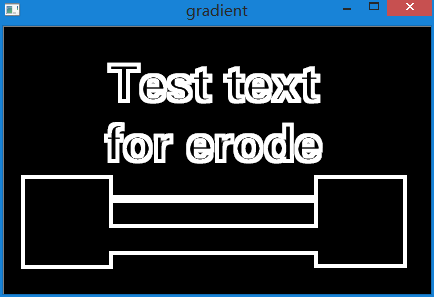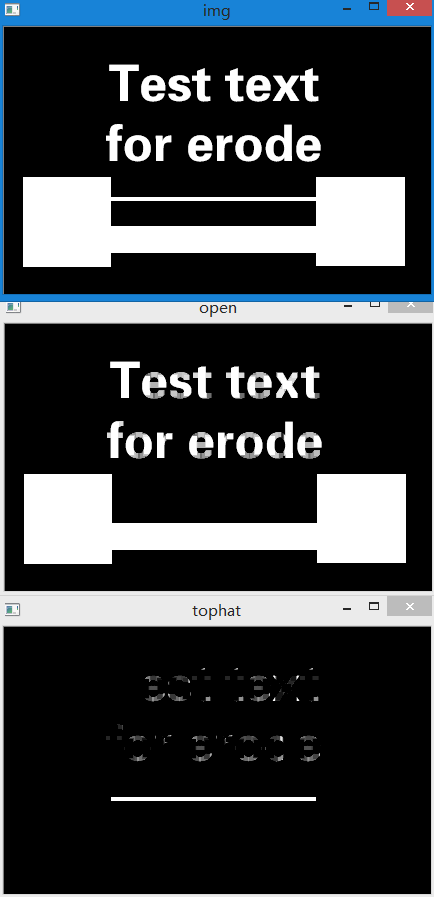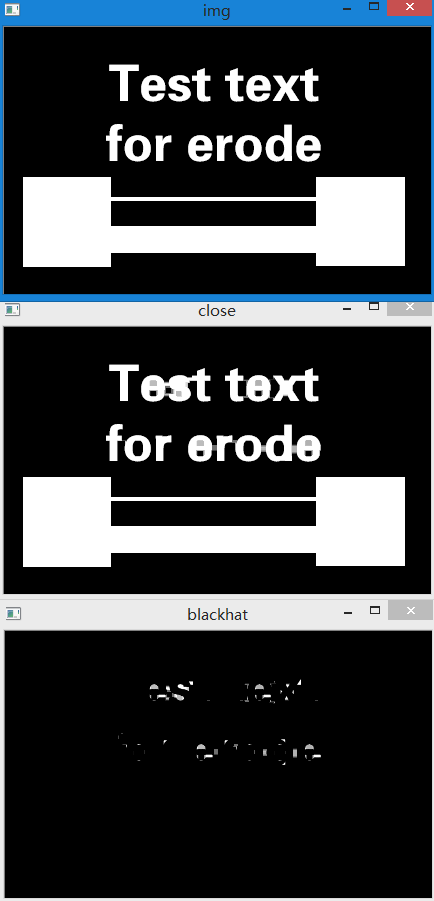# 基于Python的OpenCV图像处理7

## May 19,2017   3643 words   14 min

#### 图像形态学处理

##### 1.腐蚀

``````# coding=utf-8
import numpy as np
import cv2

# 这里我们定义了一个5×5，元素值全为1的卷积核
kernel = np.ones((5, 5), np.uint8)

# 第一个参数是待处理图像
# 第二个参数是我们定义的卷积核
# 第三个参数是腐蚀次数，可省略，默认为1
ero = cv2.erode(img, kernel, iterations=1)

cv2.imshow("img", img)
cv2.imshow("ero", ero)
cv2.waitKey(0)
``````

##### 2.膨胀

``````# coding=utf-8
import numpy as np
import cv2

# 这里我们定义了一个5×5，元素值全为1的卷积核
kernel = np.ones((5, 5), np.uint8)

# 第一个参数是待处理图像
# 第二个参数是我们定义的卷积核
# 第三个参数是膨胀次数，可省略，默认为1
dilate = cv2.dilate(img, kernel, iterations=1)

cv2.imshow("img", img)
cv2.imshow("dilate", dilate)
cv2.waitKey(0)
``````

##### 3.开运算

``````# coding=utf-8
import numpy as np
import cv2

kernel = np.ones((5, 5), np.uint8)

# 方法一 自己组合实现开运算，先腐蚀再膨胀
erode = cv2.erode(img, kernel, iterations=1)
dilate = cv2.dilate(erode, kernel, iterations=1)

# 方法二，直接使用内置函数，其中第二个参数指定的是操作类型
opening = cv2.morphologyEx(img, cv2.MORPH_OPEN, kernel)

cv2.imshow("img", img)
cv2.imshow("erode", erode)
cv2.imshow("dilate", dilate)
cv2.imshow("open", opening)
cv2.waitKey(0)

``````

##### 4.闭运算

``````# coding=utf-8
import numpy as np
import cv2

kernel = np.ones((5, 5), np.uint8)

# 方法一 自己组合实现闭运算，先膨胀再腐蚀
dilate = cv2.dilate(img, kernel, iterations=1)
erode = cv2.erode(dilate, kernel, iterations=1)

# 方法二，直接使用内置函数，其中第二个参数指定的是操作类型
close = cv2.morphologyEx(img, cv2.MORPH_CLOSE, kernel)

cv2.imshow("img", img)
cv2.imshow("erode", erode)
cv2.imshow("dilate", dilate)
cv2.imshow("close", close)
cv2.waitKey(0)
``````

##### 5.形态学梯度

``````# coding=utf-8
import numpy as np
import cv2

kernel = np.ones((5, 5), np.uint8)

# 第二个参数指定的是操作类型

cv2.waitKey(0)

``````##### 6.礼帽

``````# coding=utf-8
import numpy as np
import cv2

kernel = np.ones((5, 5), np.uint8)

# 第二个参数指定的是操作类型
open = cv2.morphologyEx(img, cv2.MORPH_OPEN, kernel)
tophat = cv2.morphologyEx(img, cv2.MORPH_TOPHAT, kernel)

cv2.imshow("img", img)
cv2.imshow("open", open)
cv2.imshow("tophat", tophat)
cv2.waitKey(0)
``````##### 7.黑帽

``````# coding=utf-8
import numpy as np
import cv2

kernel = np.ones((5, 5), np.uint8)

# 第二个参数指定的是操作类型
close = cv2.morphologyEx(img, cv2.MORPH_CLOSE, kernel)
blackhat = cv2.morphologyEx(img, cv2.MORPH_BLACKHAT, kernel)

cv2.imshow("img", img)
cv2.imshow("close", close)
cv2.imshow("blackhat", blackhat)
cv2.waitKey(0)
``````##### 8.结构化元素

``````# 普通矩形卷积核
k1 = cv2.getStructuringElement(cv2.MORPH_RECT, (5, 5))
print k1, k1.dtype

# 椭圆(圆)形卷积核
k2 = cv2.getStructuringElement(cv2.MORPH_ELLIPSE, (5, 5))
print k2, k2.dtype

# 十字形卷积核
k3 = cv2.getStructuringElement(cv2.MORPH_CROSS, (5, 5))
print k3, k3.dtype
``````

``````[[1 1 1 1 1]
[1 1 1 1 1]
[1 1 1 1 1]
[1 1 1 1 1]
[1 1 1 1 1]] uint8

[[0 0 1 0 0]
[1 1 1 1 1]
[1 1 1 1 1]
[1 1 1 1 1]
[0 0 1 0 0]] uint8

[[0 0 1 0 0]
[0 0 1 0 0]
[1 1 1 1 1]
[0 0 1 0 0]
[0 0 1 0 0]] uint8
``````